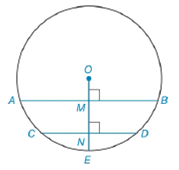Chapter 6.4, Problem 39EElementary Geometry For College St...

7th Edition
Alexander + 2 others
ISBN: 9781337614085

Solutions

Chapter
SectionElementary Geometry For College St...

7th Edition
Alexander + 2 others
ISBN: 9781337614085
Textbook Problem

In ⊙ O , chord A B ¯ ∥ chord C D ¯ . Radius O E ¯ is perpendicular to A B ¯ and C D ¯ at points M and N , respectively. If O E = 13 , A B = 24 , and C D = 10 , then the distance from O to C D ¯ is greater than the distance from O to A B ¯ . Determine how much farther chord C D ¯ is from center O than chord A B ¯ is from center O ; that is, find M N .To determine

To find:

The distance chord CD¯ is farther from center O than chord AB¯ is from center O; that is, find MN.

Explanation

Given:

O, chord AB¯ chord CD¯. Radius OE¯ is perpendicular to AB¯ and CD¯ at points M and N, respectively. OE=13, AB=24, and CD=10, such that the distance from O to CD¯ is greater than the distance from O to AB¯.

The figure given below,

Theorem Used:

According to the Pythagorean theorem, in a right-angled triangle,

hypotenuse2=base2+perpendicular2.

Calculation:

Since the chords AB=24, and CD=10 are unequal i.e. mCD¯>mAB¯ and the distance from O to CD¯ is greater than the distance from O to AB¯ i.e. mON¯>mOM¯ and applying Pythagorean theorem from the figure below,

In AOB,

OB¯2=OM¯2+MB¯2 or OM¯=OB¯2MB¯2

And from COD

OC¯2=ON¯2+NC¯2 or ON¯=OC¯2NC¯2

Since OM and ON are perpendicular bisector as a radius perpendicular to a chord bisects the chord and its arc

Still sussing out bartleby?

Check out a sample textbook solution.

See a sample solution

The Solution to Your Study Problems

Bartleby provides explanations to thousands of textbook problems written by our experts, many with advanced degrees!

Get Started

Find for the functions in Problems 7-10. 8.

Mathematical Applications for the Management, Life, and Social Sciences

Subtract and check: 4000702_

Elementary Technical Mathematics

In Exercises 6372, evaluate the expression. 64. 4 + |4|

Applied Calculus for the Managerial, Life, and Social Sciences: A Brief Approach

Find all possible real solutions of each equation in Exercises 3144. x3x25x+5=0

Finite Mathematics and Applied Calculus (MindTap Course List)

Determine whether f'(0) exists. f(x)={x2sin1xifx00ifx=0

Single Variable Calculus: Early Transcendentals, Volume I

The arc length of y = 3x + 2 from x = 1 to x = 5 is a) 210 b) 52 c) 102 d) 410

Study Guide for Stewart's Single Variable Calculus: Early Transcendentals, 8th